# Elementary Particles Lecture 4 Niels Tuning Harry van

• Slides: 89
Download presentation“Elementary Particles” Lecture 4 Niels Tuning Harry van der Graaf Niels Tuning (1)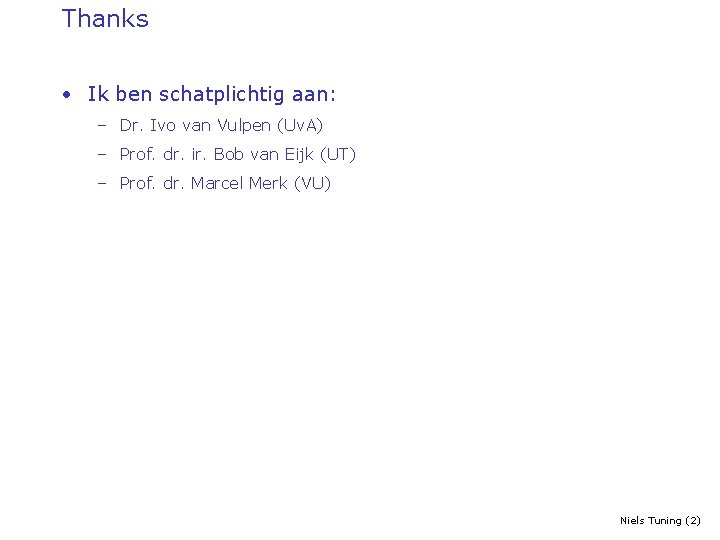Thanks • Ik ben schatplichtig aan: – Dr. Ivo van Vulpen (Uv. A) – Prof. dr. ir. Bob van Eijk (UT) – Prof. dr. Marcel Merk (VU) Niels Tuning (2)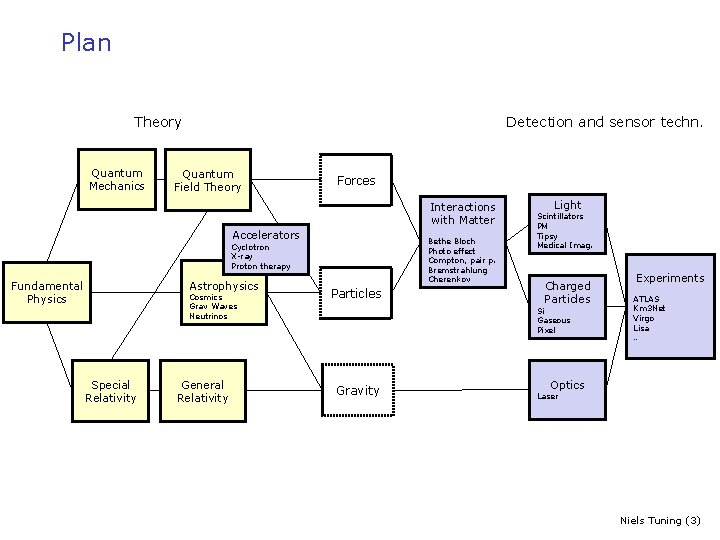Plan Theory Quantum Mechanics Detection and sensor techn. Quantum Field Theory Forces Interactions with Matter Accelerators Bethe Bloch Photo effect Compton, pair p. Bremstrahlung Cherenkov Cyclotron X-ray Proton therapy Astrophysics Fundamental Physics Cosmics Grav Waves Neutrinos Special Relativity General Relativity Particles Light Scintillators PM Tipsy Medical Imag. Charged Particles Si Gaseous Pixel Gravity Experiments ATLAS Km 3 Net Virgo Lisa … Optics Laser Niels Tuning (3)Plan Today pe 2) Niels Quantum Field Theory sw ap 2) Niels Quantum Mechanics d Theory Fundamental Physics 6) Ernst-Jan Niels 7) + 10) Forces 5) + 8) Particles 1) Harry Accelerators Astrophysics 1) Niels Special Relativity 9) Ernst-Jan General Relativity Detection and sensor techn. 3) Harry Relativistic. I nteractions with Matter 4) Harry Light 11) +12) Martin Charged Particles 9) Ernst-Jan Gravity 6) + 9) Ernst-Jan Martin 13) + 14) Excursions Experiments 9) Ernst-Jan Optics Niels Tuning (4)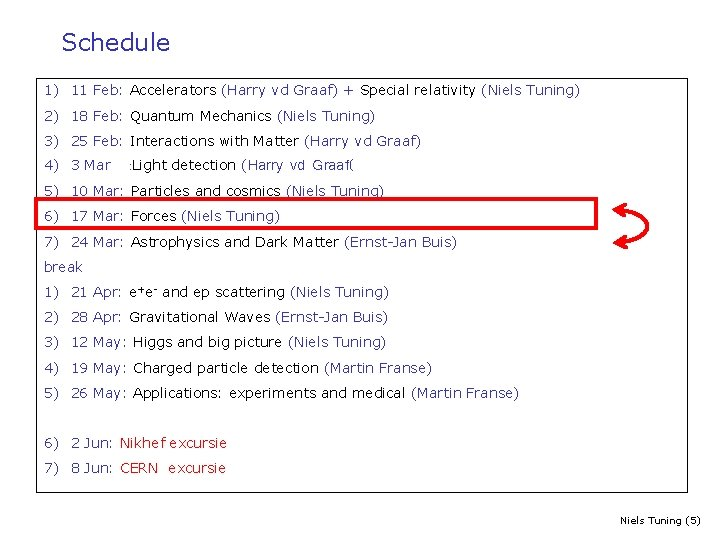Schedule 1) 11 Feb: Accelerators (Harry vd Graaf) + Special relativity (Niels Tuning) 2) 18 Feb: Quantum Mechanics (Niels Tuning) 3) 25 Feb: Interactions with Matter (Harry vd Graaf) 4) 3 Mar : Light detection (Harry vd Graaf( 5) 10 Mar: Particles and cosmics (Niels Tuning) 6) 17 Mar: Forces (Niels Tuning) 7) 24 Mar: Astrophysics and Dark Matter (Ernst-Jan Buis) break 1) 21 Apr: e+e- and ep scattering (Niels Tuning) 2) 28 Apr: Gravitational Waves (Ernst-Jan Buis) 3) 12 May: Higgs and big picture (Niels Tuning) 4) 19 May: Charged particle detection (Martin Franse) 5) 26 May: Applications: experiments and medical (Martin Franse) 6) 2 Jun: Nikhef excursie 7) 8 Jun: CERN excursie Niels Tuning (5)Plan 1900 -1940 1945 -1965 -1975 1) Intro: Standard Model & Relativity 11 Feb 2) Basis 18 Feb 1) Atom model, strong and weak force 2) Scattering theory 3) Hadrons 1) Isospin, strangeness 2) Quark model, GIM 4) Standard Model 1) QED 2) Parity, neutrinos, weak inteaction 3) QCD 10 Mar 17 Mar 1975 -2000 5) e+e- and DIS 21 Apr 2000 -2015 6) Higgs and CKM 12 May Niels Tuning (6)Homework 1) Homework for this and previous lecture 2) Hand in before 21 AprilOutline for today: Interactions 1) Gauge invariance, and the Lagrangian 2) Electro-magnetic interaction § QED 3) Weak interaction § Parity violation 4) Strong interaction § QCD Niels Tuning (8)Summary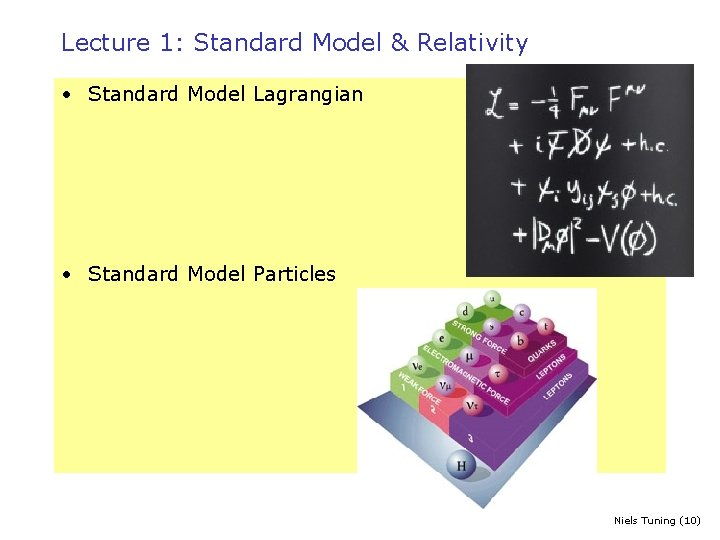Lecture 1: Standard Model & Relativity • Standard Model Lagrangian • Standard Model Particles Niels Tuning (10)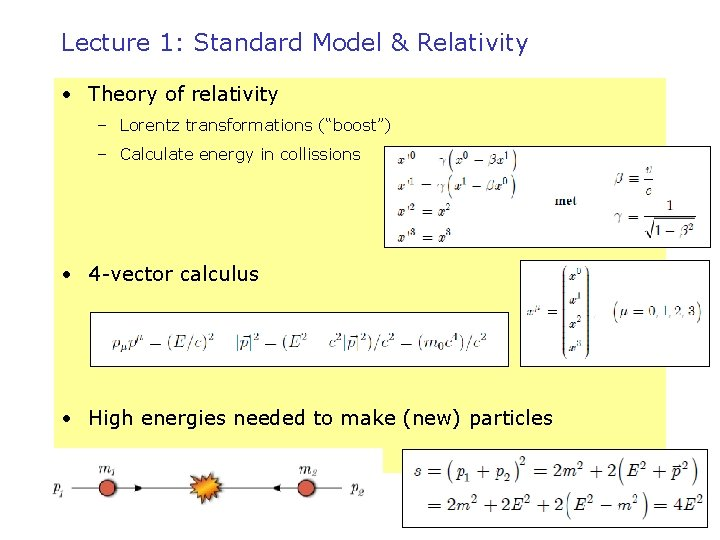Lecture 1: Standard Model & Relativity • Theory of relativity – Lorentz transformations (“boost”) – Calculate energy in collissions • 4 -vector calculus • High energies needed to make (new) particles Niels Tuning (11)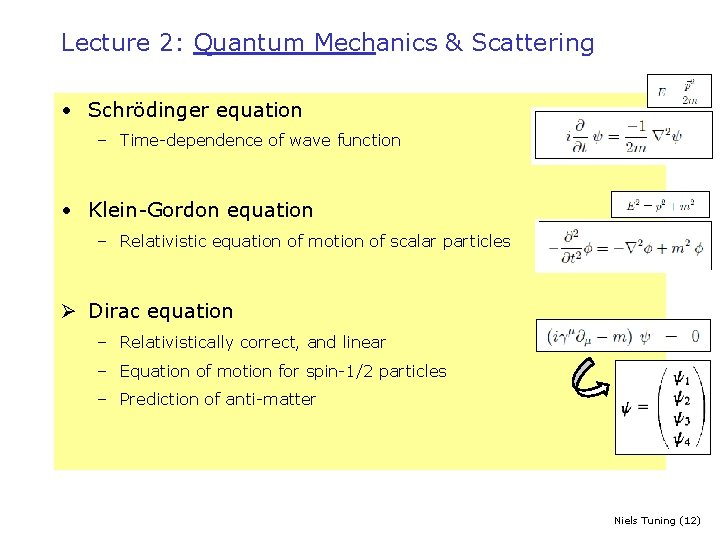Lecture 2: Quantum Mechanics & Scattering • Schrödinger equation – Time-dependence of wave function • Klein-Gordon equation – Relativistic equation of motion of scalar particles Ø Dirac equation – Relativistically correct, and linear – Equation of motion for spin-1/2 particles – Prediction of anti-matter Niels Tuning (12)Lecture 2: Quantum Mechanics & Scattering • Scattering Theory – (Relative) probability for certain process to happen – Cross section Scattering amplitude in Quantum Field Theory Classic • Fermi’s Golden Rule – Decay: “decay width” Γ a→b+c – Scattering: “cross section” σ a+b→c+d Niels Tuning (13)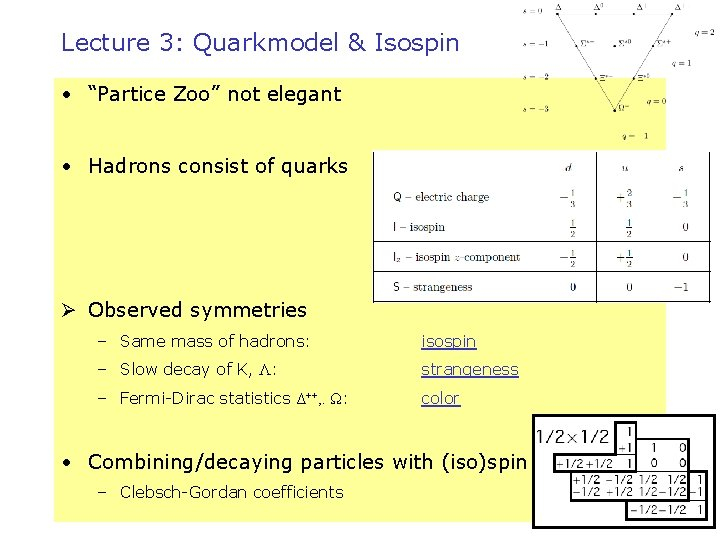Lecture 3: Quarkmodel & Isospin • “Partice Zoo” not elegant • Hadrons consist of quarks Ø Observed symmetries – Same mass of hadrons: isospin – Slow decay of K, Λ: strangeness – Fermi-Dirac statistics Δ++, . Ω: color • Combining/decaying particles with (iso)spin – Clebsch-Gordan coefficients Niels Tuning (14)Group theory • Mesons: – 2 quarks, with 3 possible flavours: u, d, s – 32 =9 possibilities = 8 + 1 q=1 Niels Tuning (15)Group theory • Mesons: – 2 quarks, with 3 possible flavours: u, d, s – 32 =9 possibilities = 8 + 1 η' Niels Tuning (16)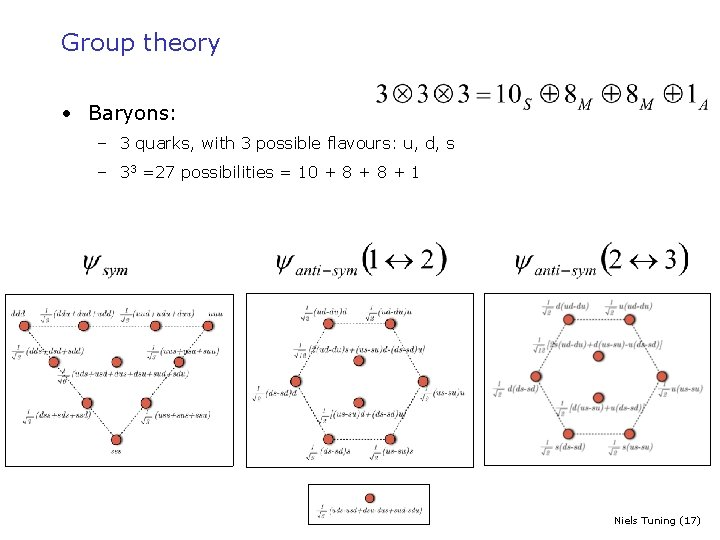Group theory • Baryons: – 3 quarks, with 3 possible flavours: u, d, s – 33 =27 possibilities = 10 + 8 + 1 Niels Tuning (17)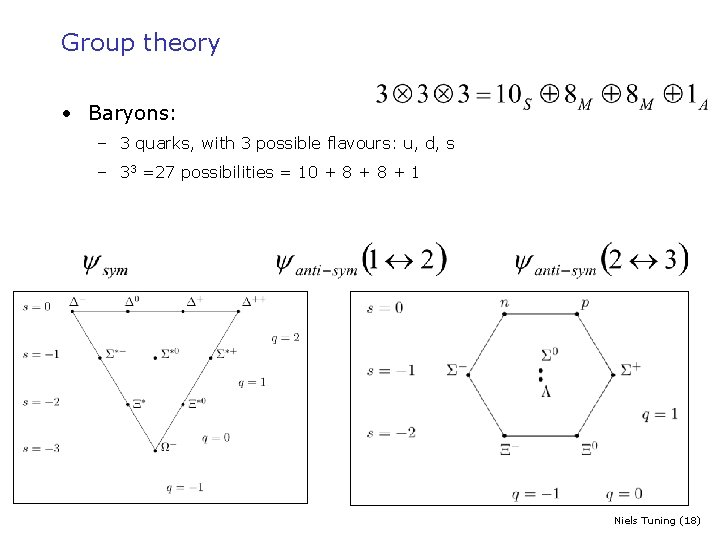Group theory • Baryons: – 3 quarks, with 3 possible flavours: u, d, s – 33 =27 possibilities = 10 + 8 + 1 Niels Tuning (18)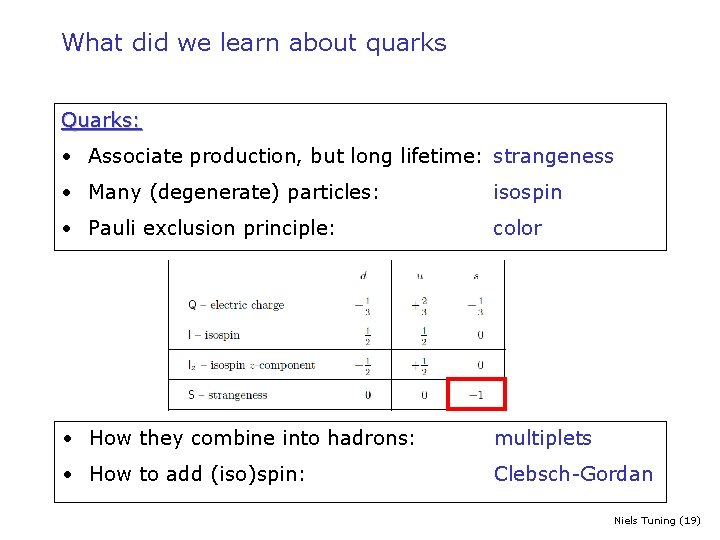What did we learn about quarks Quarks: • Associate production, but long lifetime: strangeness • Many (degenerate) particles: isospin • Pauli exclusion principle: color • How they combine into hadrons: multiplets • How to add (iso)spin: Clebsch-Gordan Niels Tuning (19)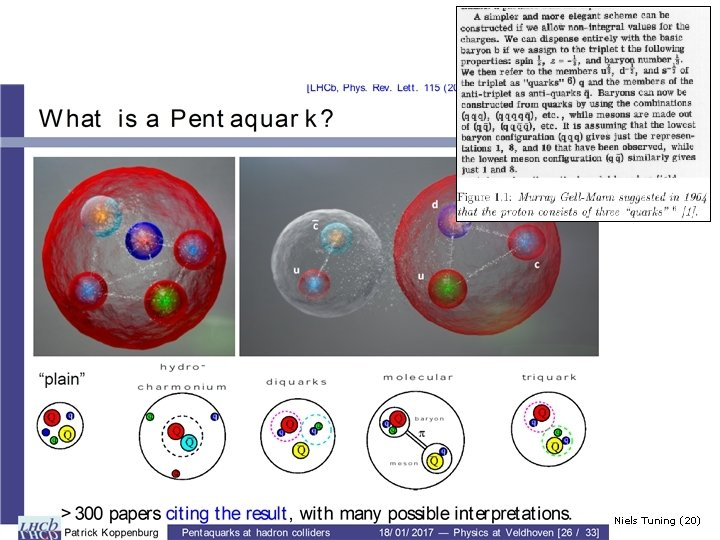Niels Tuning (20)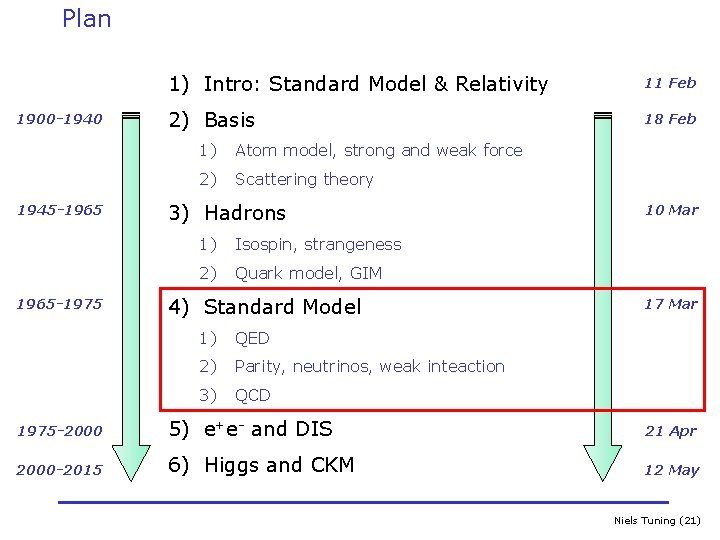Plan 1900 -1940 1945 -1965 -1975 1) Intro: Standard Model & Relativity 11 Feb 2) Basis 18 Feb 1) Atom model, strong and weak force 2) Scattering theory 3) Hadrons 1) Isospin, strangeness 2) Quark model, GIM 4) Standard Model 1) QED 2) Parity, neutrinos, weak inteaction 3) QCD 10 Mar 17 Mar 1975 -2000 5) e+e- and DIS 21 Apr 2000 -2015 6) Higgs and CKM 12 May Niels Tuning (21)Model elementary particles Tools Cross section Kinematic s Detectors Atom Strong force Mesons Particle Physics Leptons Quark model Baryons Quantum numbers Conserva tion Laws Standard Model Particles quarks/leptons Accele rators Theory of Relativity Quantum mechanic s Forces Electromagnetic Weak Strong Group Theory Quantumfield theory & Local gauge invarianceLecture 4: Forces Niels Tuning (23)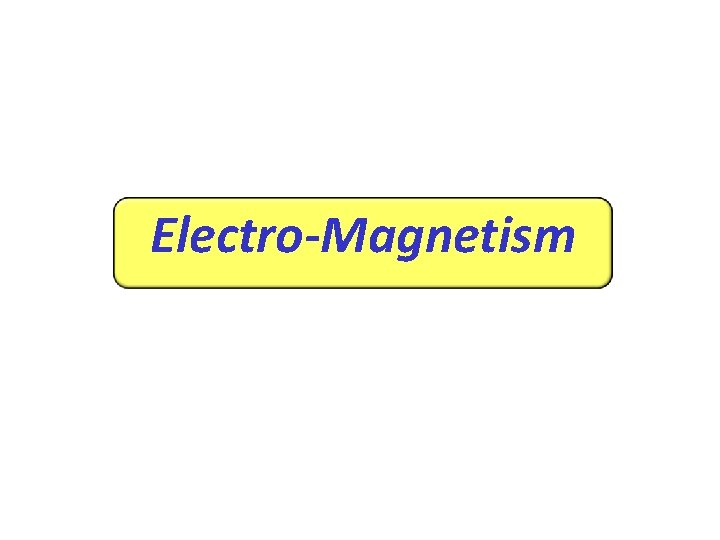Electro-Magnetism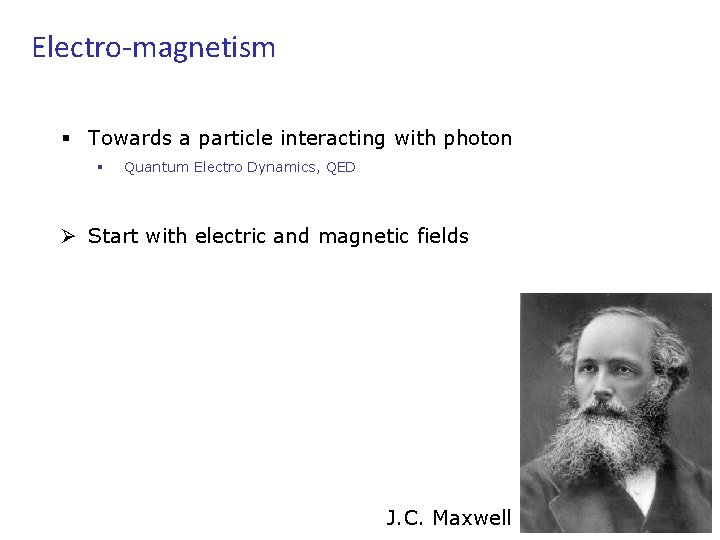Electro-magnetism § Towards a particle interacting with photon § Quantum Electro Dynamics, QED Ø Start with electric and magnetic fields J. C. Maxwell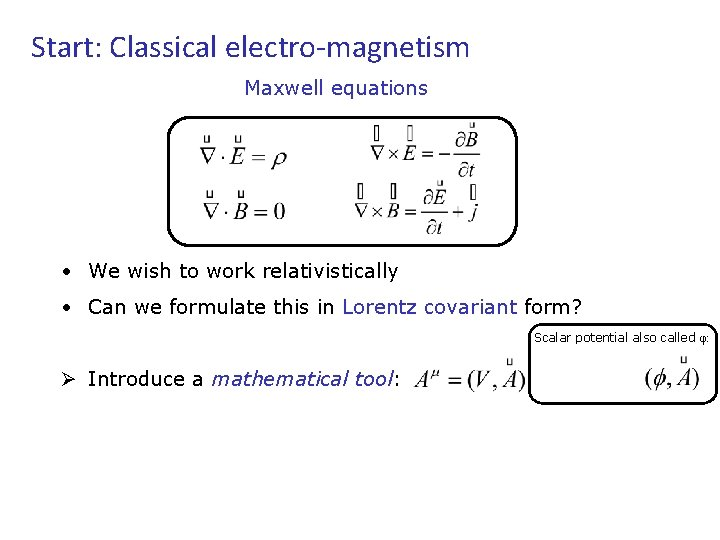Start: Classical electro-magnetism Maxwell equations • We wish to work relativistically • Can we formulate this in Lorentz covariant form? Scalar potential also called φ: Ø Introduce a mathematical tool:Start: Classical electro-magnetism Maxwell equations • We wish to work relativistically • Can we formulate this in Lorentz covariant form? Scalar potential also called φ: • Introduce a mathematical tool: • Note: Ø Choose: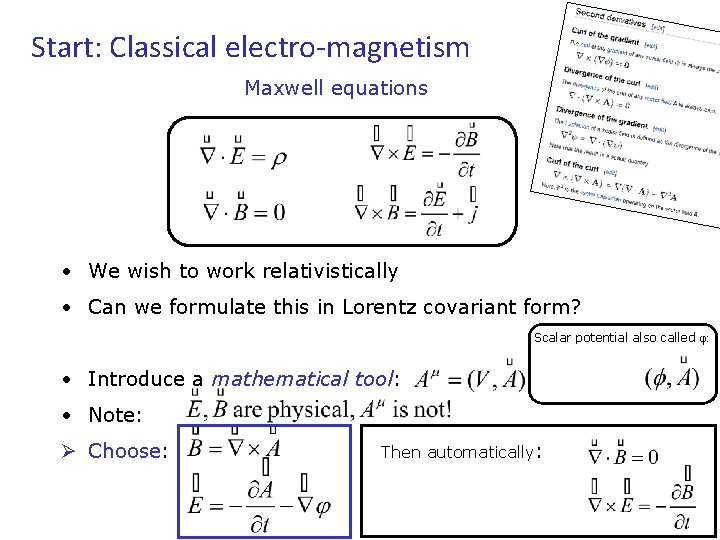Start: Classical electro-magnetism Maxwell equations • We wish to work relativistically • Can we formulate this in Lorentz covariant form? Scalar potential also called φ: • Introduce a mathematical tool: • Note: Ø Choose: Then automatically:Rewrite Maxwell equations • Maxwell eqs. can then be written quite economically…: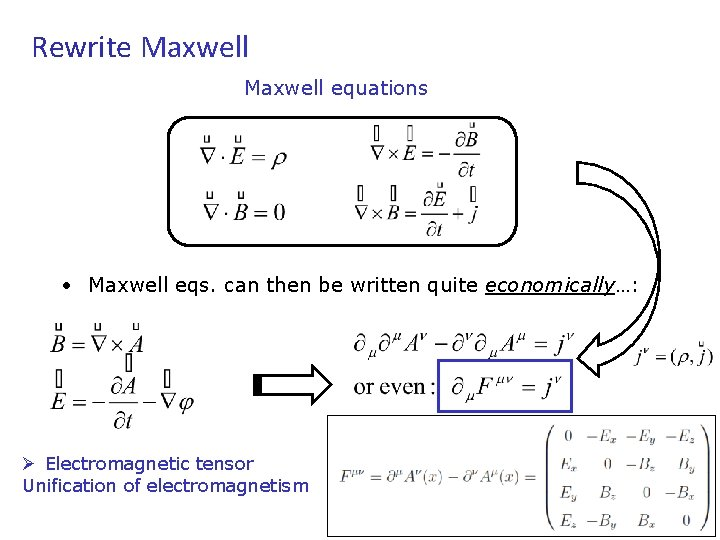Rewrite Maxwell equations • Maxwell eqs. can then be written quite economically…: Ø Electromagnetic tensor Unification of electromagnetismGauge Invariance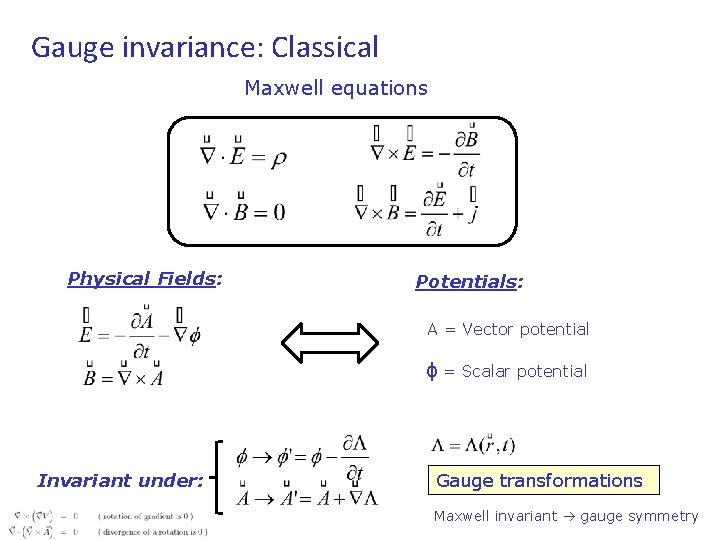Gauge invariance: Classical Maxwell equations Physical Fields: Potentials: A = Vector potential ϕ = Scalar potential Invariant under: Gauge transformations Maxwell invariant gauge symmetryGauge invariance: Classical Maxwell equations Physical Fields: Potentials: A = Vector potential ϕ = Scalar potential Invariant under: Gauge transformations Maxwell invariant gauge symmetryNot unique! Can choose extra constraints: Coulomb-gauge: Lorenz-gauge: Advantage: Lorentz-invariant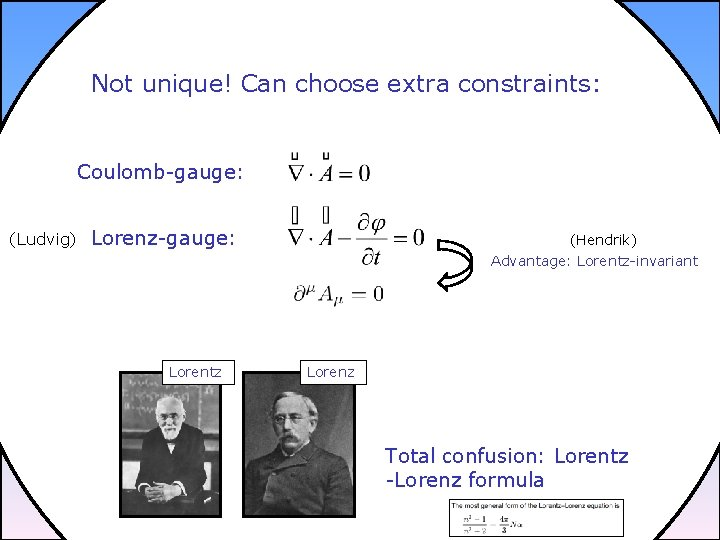Not unique! Can choose extra constraints: Coulomb-gauge: (Ludvig) Lorenz-gauge: (Hendrik) Advantage: Lorentz-invariant Lorentz Lorenz Total confusion: Lorentz -Lorenz formula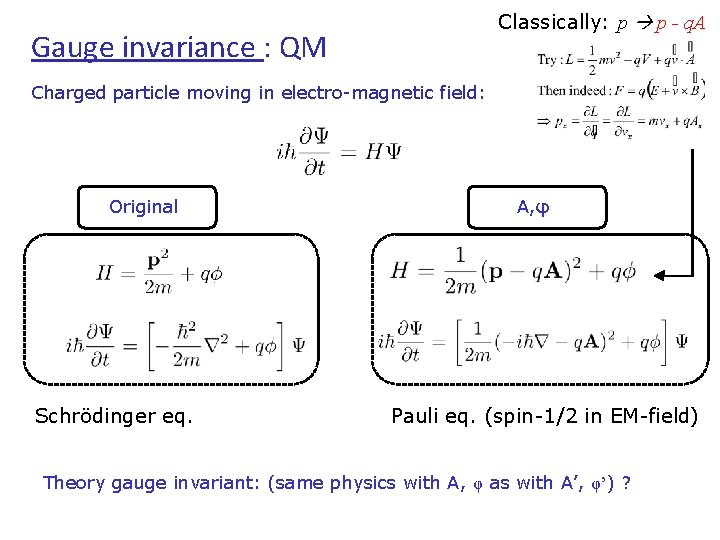Classically: p p - q. A Gauge invariance : QM Charged particle moving in electro-magnetic field: Original Schrödinger eq. A, φ Pauli eq. (spin-1/2 in EM-field) Theory gauge invariant: (same physics with A, φ as with A’, φ’) ?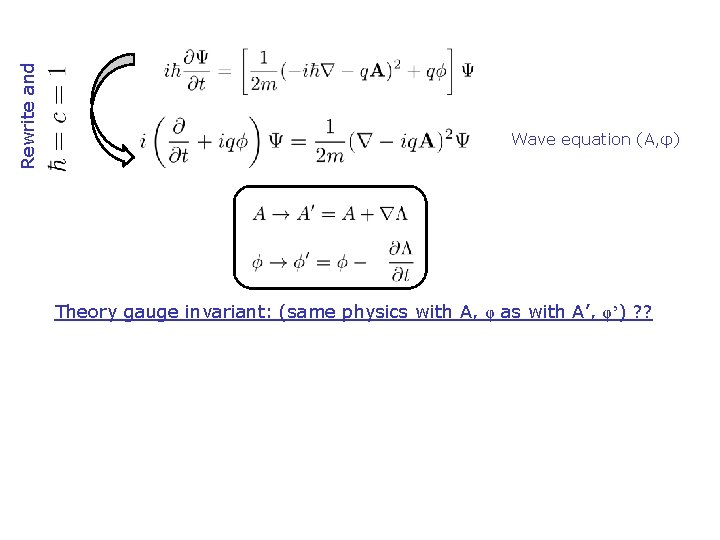Rewrite and Wave equation (A, φ) Theory gauge invariant: (same physics with A, φ as with A’, φ’) ? ?Rewrite and Wave equation (A, φ) Theory gauge invariant: (same physics with A, φ as with A’, φ’) ? ? Wave equation (A’, φ’) Yes! IfLocal gauge symmetry Schrödinger equation (time-independent): Global phase: Ψ’ stays solution of Schrödinger eq!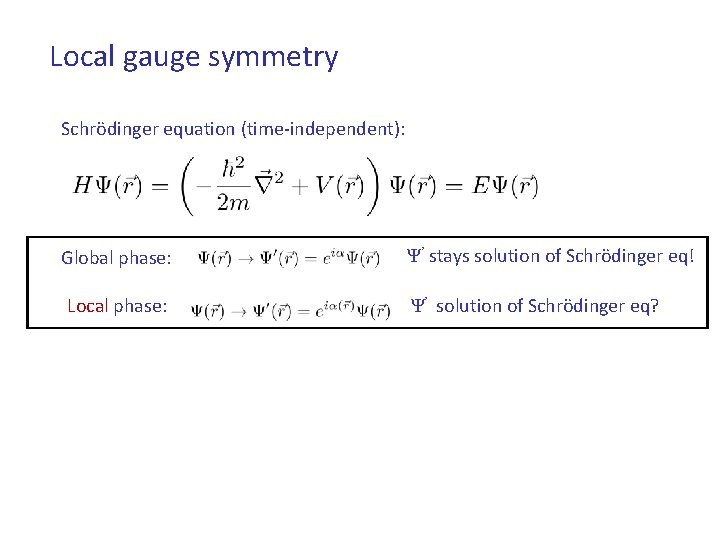Local gauge symmetry Schrödinger equation (time-independent): Global phase: Ψ’ stays solution of Schrödinger eq! Local phase: Ψ’ solution of Schrödinger eq?Local gauge symmetry Schrödinger equation (time-independent): Global phase: Ψ’ stays solution of Schrodinger eq! Local phase: Ψ’ solution of Schrodinger eq? No: Ø (How) can you keep the Schrödinger equation invariant ?Before going to Quantum Field Theory, lets remind ourselves of the Lagrange formalism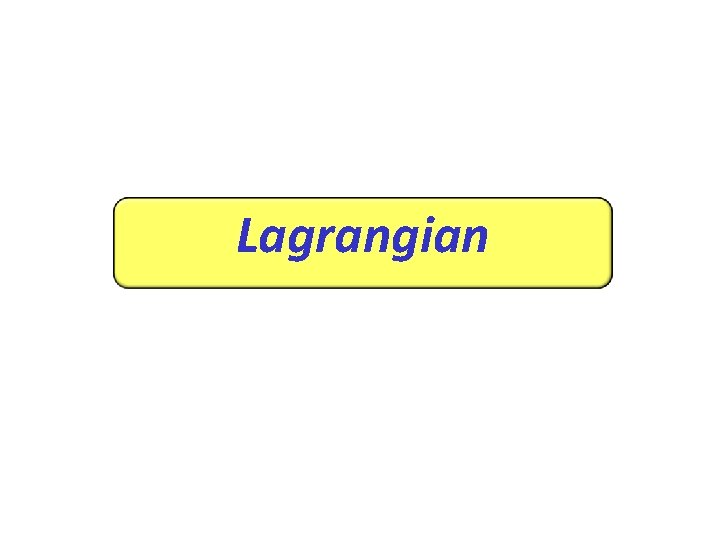LagrangianEuler-Lagrange • Euler-Lagrange equations: • Least-action, or Hamilton’s principle: Niels Tuning (44)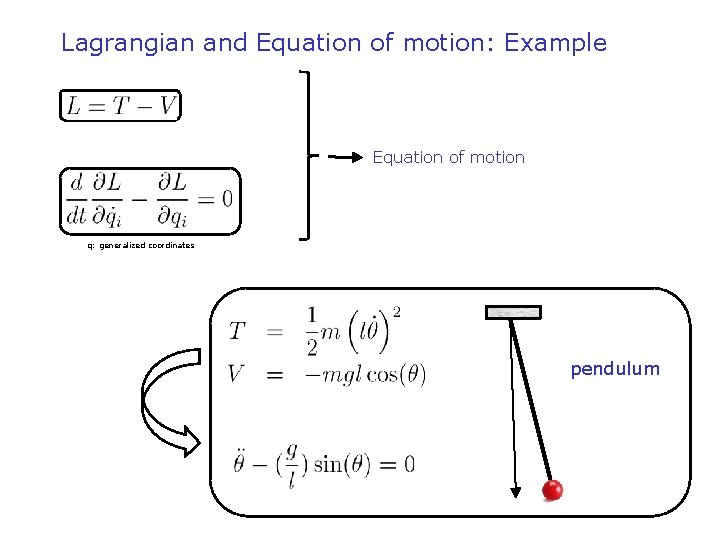Lagrangian and Equation of motion: Example 1) Lagrangiaan 2) Euler-Lagrange vergelijking Equation of motion q: generalized coordinates pendulum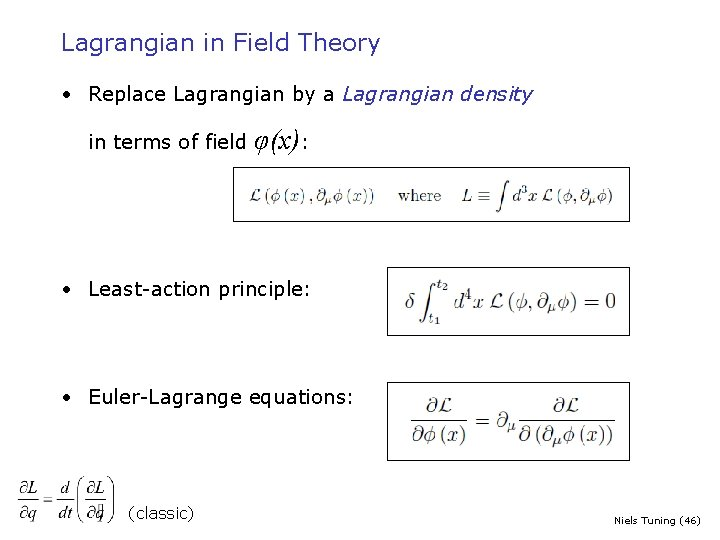Lagrangian in Field Theory • Replace Lagrangian by a Lagrangian density in terms of field φ(x): • Least-action principle: • Euler-Lagrange equations: (classic) Niels Tuning (46)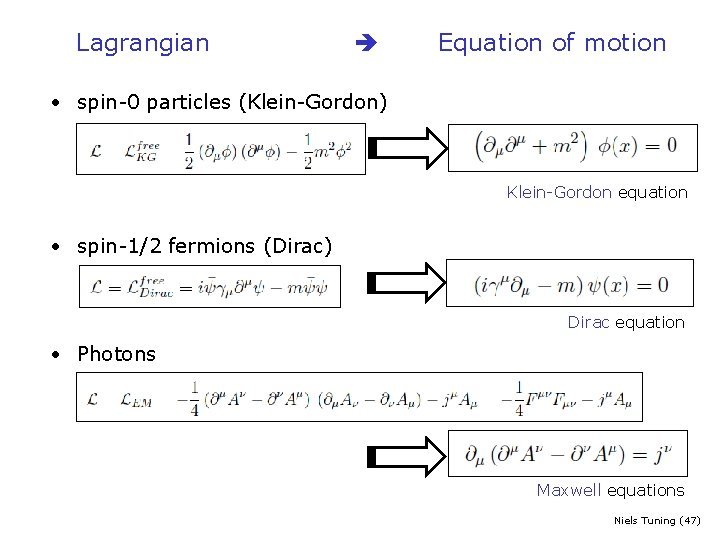Lagrangian Equation of motion • spin-0 particles (Klein-Gordon) Klein-Gordon equation • spin-1/2 fermions (Dirac) Dirac equation • Photons Maxwell equations Niels Tuning (47)QED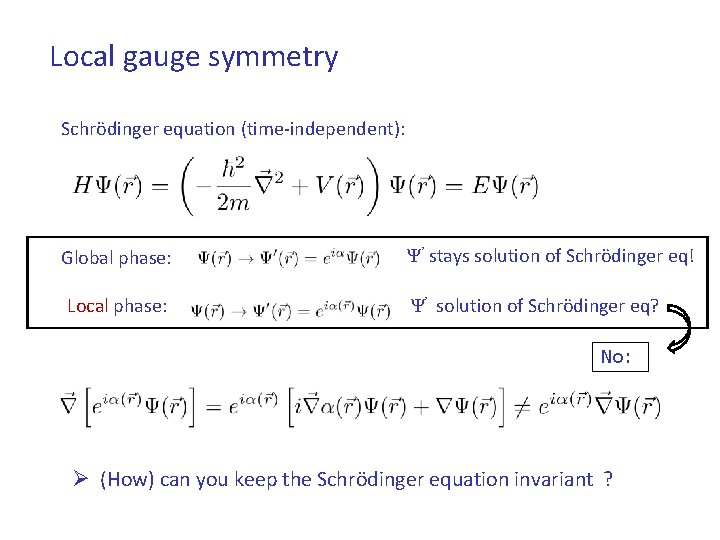Local gauge symmetry Schrödinger equation (time-independent): Global phase: Ψ’ stays solution of Schrödinger eq! Local phase: Ψ’ solution of Schrödinger eq? No: Ø (How) can you keep the Schrödinger equation invariant ?Phase Invariance • Quantum Mechanics: – Expectation value: – Observation is invariant under transformation: • But what happens to the Lagrangian density, ? – Depends also on derivative. . . , so: Niels Tuning (50)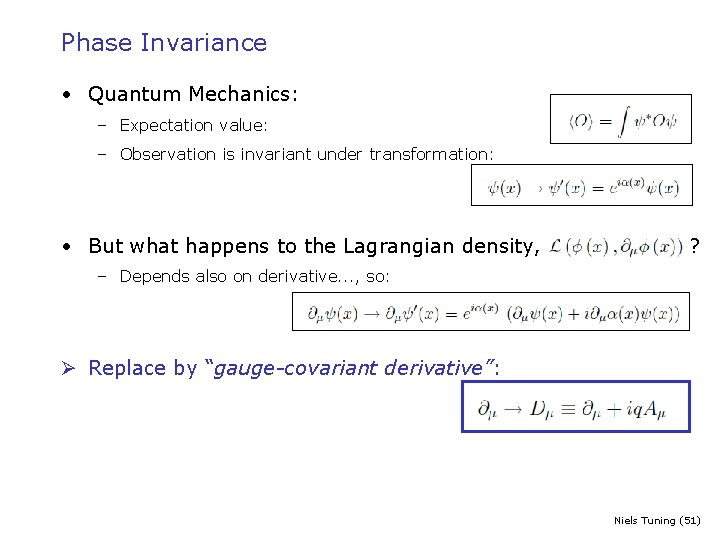Phase Invariance • Quantum Mechanics: – Expectation value: – Observation is invariant under transformation: • But what happens to the Lagrangian density, ? – Depends also on derivative. . . , so: Ø Replace by “gauge-covariant derivative”: Niels Tuning (51)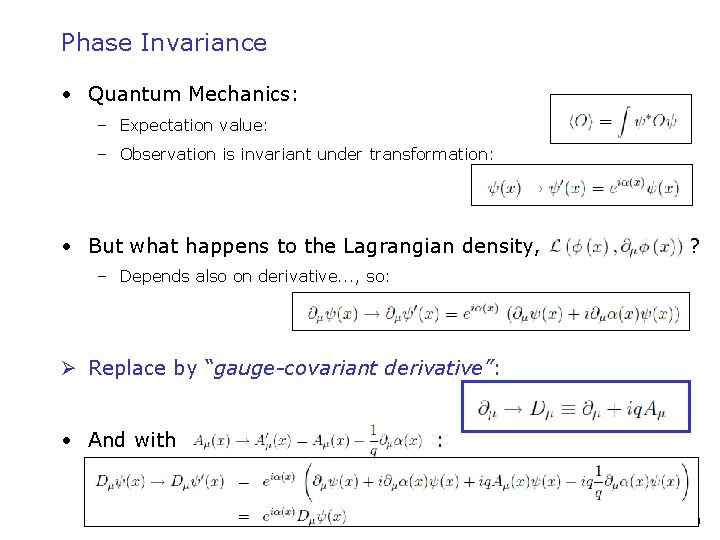Phase Invariance • Quantum Mechanics: – Expectation value: – Observation is invariant under transformation: • But what happens to the Lagrangian density, ? – Depends also on derivative. . . , so: Ø Replace by “gauge-covariant derivative”: • And with : Niels Tuning (52)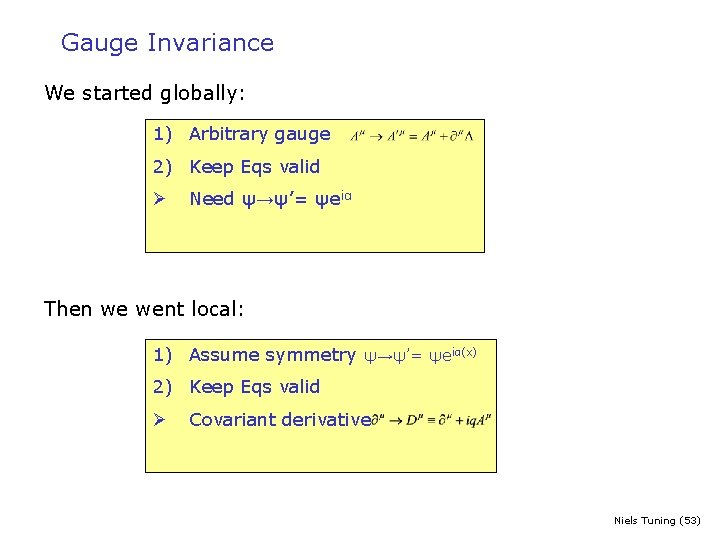Gauge Invariance We started globally: 1) Arbitrary gauge 2) Keep Eqs valid Ø Need ψ→ψ’= ψeiα Then we went local: 1) Assume symmetry ψ→ψ’= ψeiα(x) 2) Keep Eqs valid Ø Covariant derivative Niels Tuning (53)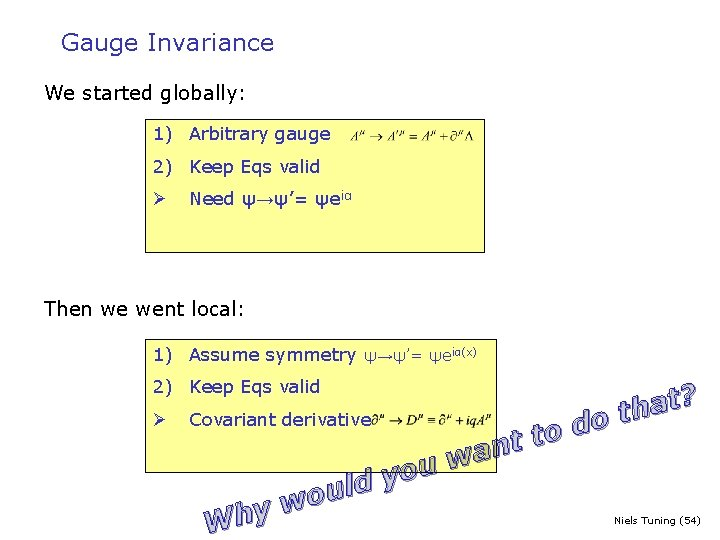Gauge Invariance We started globally: 1) Arbitrary gauge 2) Keep Eqs valid Ø Need ψ→ψ’= ψeiα Then we went local: 1) Assume symmetry ψ→ψ’= ψeiα(x) 2) Keep Eqs valid Ø ? t a h t Covariant derivative o d o t t an w u o y ld u o w y h W Niels Tuning (54)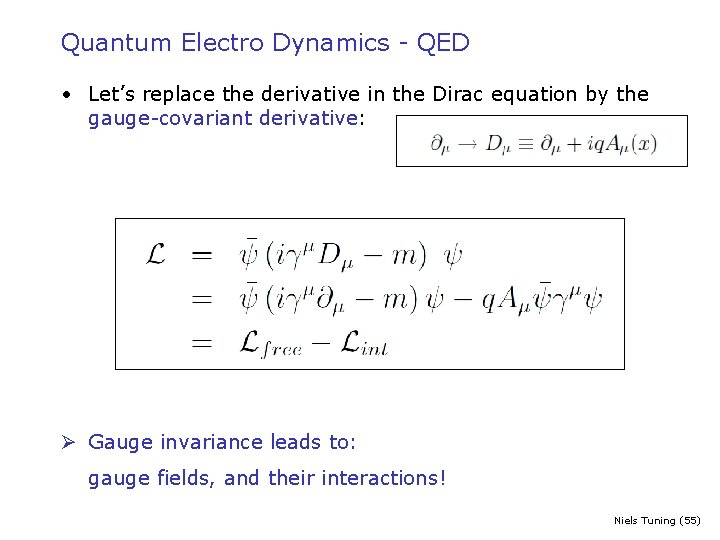Quantum Electro Dynamics - QED • Let’s replace the derivative in the Dirac equation by the gauge-covariant derivative: Ø Gauge invariance leads to: gauge fields, and their interactions! Niels Tuning (55)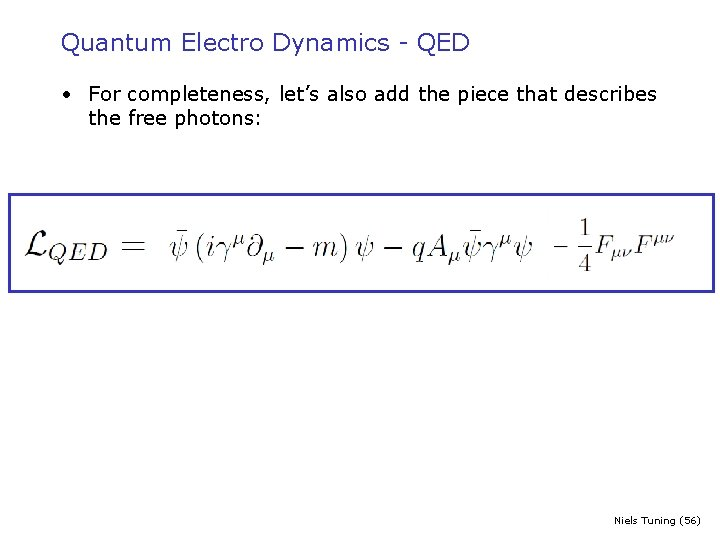Quantum Electro Dynamics - QED • For completeness, let’s also add the piece that describes the free photons: Niels Tuning (56)Quantum Electro Dynamics - QED Niels Tuning (57)Quantum Electro Dynamics - QED m Fermion ψ with mass m qγμ Interaction with coupling q Photon field Aμ Niels Tuning (58)Local gauge symmetry Fundamental symmetry Basis of forces in Standard Model and Forces arise beautifully! Gauge field(+ interactions)Local gauge symmetry Pragmatic: We have forces and Basis of forces in Standard Model Can be described mathematically Gauge field(+ interactions)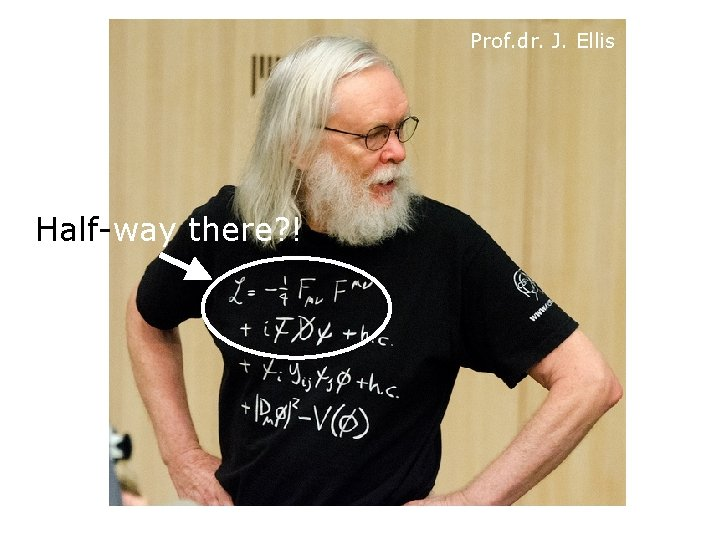Prof. dr. J. Ellis Half-way there? !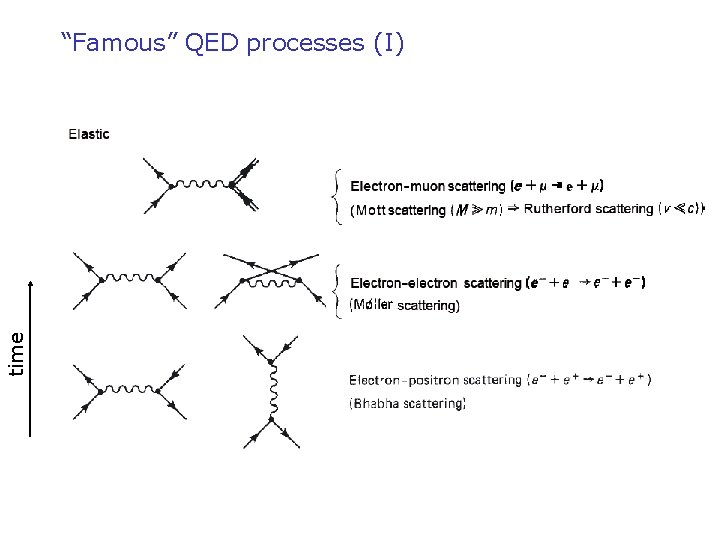time “Famous” QED processes (I)time “Famous” QED processes (II)Weak Interaction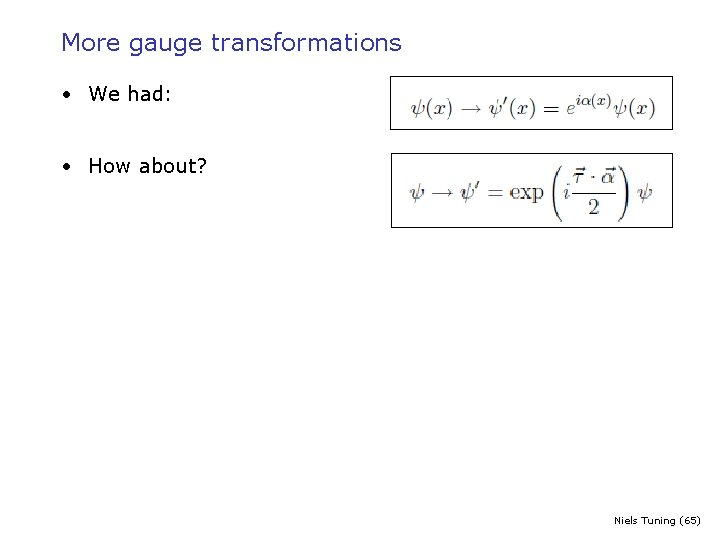More gauge transformations • We had: • How about? Niels Tuning (65)More gauge transformations • We had: • How about? • Why? Historical origin: proton and neutron exhibit isospin symmetry Niels Tuning (66)More gauge transformations • We had: • How about? • Introduce new covariant derivative: • Bμ is now 2 x 2 matrix: Ø with three gauge fields: Niels Tuning (67)Electro-weak theory • The three b, are associated to the W+, W- and Z 0 Niels Tuning (68)Electroweak theory Ø We measured that left and right are different! • Instead of “strong” isospin, switch to “weak” isospin: • Formalism the same (What is difference between chirality and helicity…? ) Niels Tuning (69)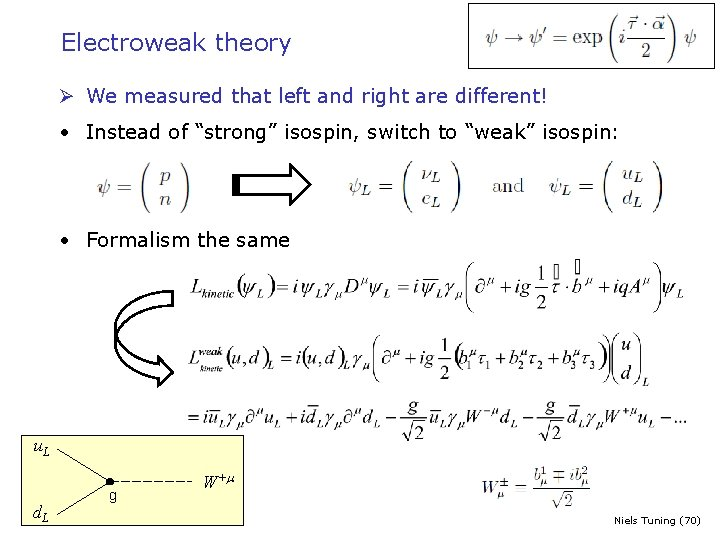Electroweak theory Ø We measured that left and right are different! • Instead of “strong” isospin, switch to “weak” isospin: • Formalism the same u. L d. L g W+m Niels Tuning (70)Parity violation • 1956: – C. -S. Wu discovered that neutrino’s are always left-handed – The process involved: – 60 Co 27 is spin-5 and 60 Ni 28 60 27 Co 60 28 Ni + e- + ne is spin-4, both e- and ne are spin-½ n-e 60 Co e- Ø All neutrino’s are left-handed Ø All antineutrinos are right-handed Niels Tuning (71)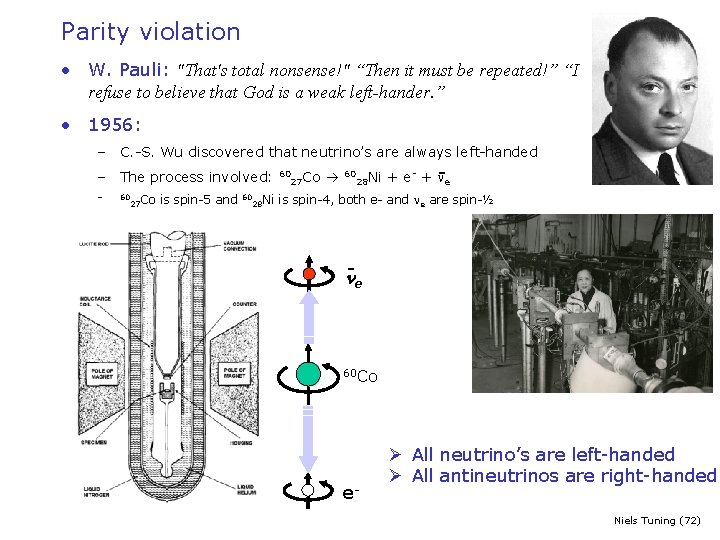Parity violation • W. Pauli: "That's total nonsense!" “Then it must be repeated!” “I refuse to believe that God is a weak left-hander. ” • 1956: – C. -S. Wu discovered that neutrino’s are always left-handed – The process involved: – 60 Co 27 is spin-5 and 60 Ni 28 60 27 Co 60 28 Ni + e- + ne is spin-4, both e- and ne are spin-½ n-e 60 Co e- Ø All neutrino’s are left-handed Ø All antineutrinos are right-handed Niels Tuning (72)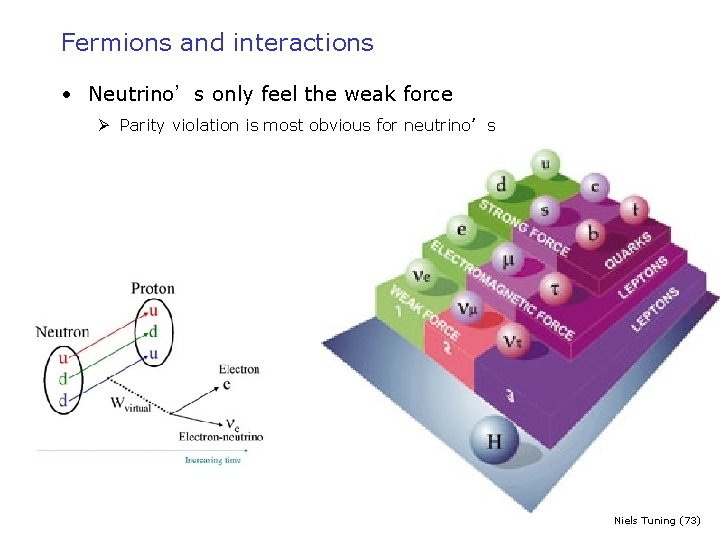Fermions and interactions • Neutrino’s only feel the weak force Ø Parity violation is most obvious for neutrino’s Niels Tuning (73)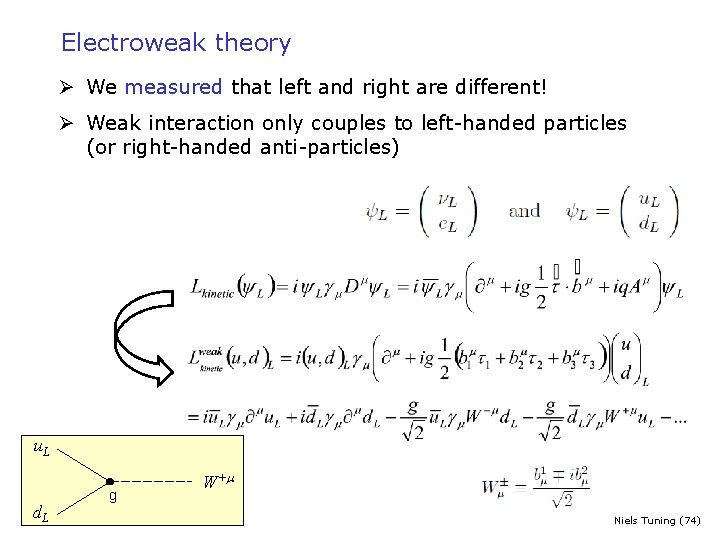Electroweak theory Ø We measured that left and right are different! Ø Weak interaction only couples to left-handed particles (or right-handed anti-particles) u. L d. L g W+m Niels Tuning (74)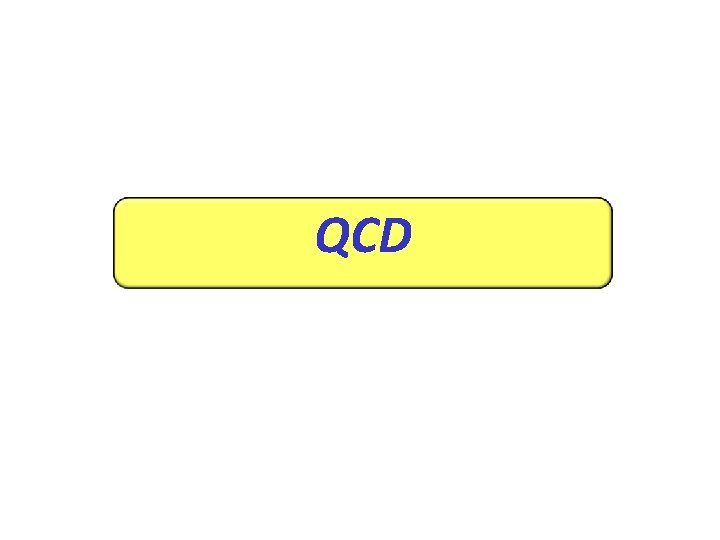QCDSymmetries • Charge • Isospin • Color Niels Tuning (76)More gauge transformations • We had: U(1) • Then: SU(2) (Weak) • How about: SU(3) (QED) (QCD) (Why 8…? Group theory: 3 x 3=8+1 … ) Niels Tuning (77)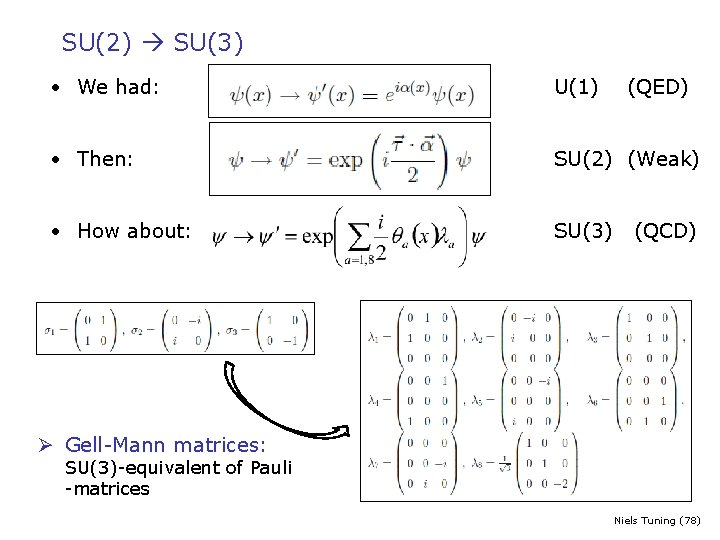SU(2) SU(3) • We had: U(1) • Then: SU(2) (Weak) • How about: SU(3) (QED) (QCD) Ø Gell-Mann matrices: SU(3)-equivalent of Pauli -matrices Niels Tuning (78)Symmetries • Charge U(1) • Isospin SU(2) (Weak) • Color SU(3) (QED) (QCD) (Why 8…? Group theory: 3 x 3=8+1 … ) Niels Tuning (79)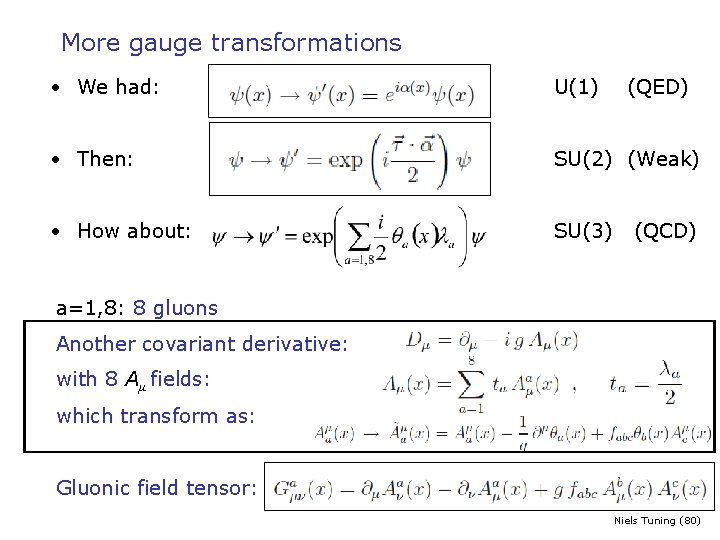More gauge transformations • We had: U(1) • Then: SU(2) (Weak) • How about: SU(3) (QED) (QCD) a=1, 8: 8 gluons Another covariant derivative: with 8 Aμ fields: which transform as: Gluonic field tensor: Niels Tuning (80)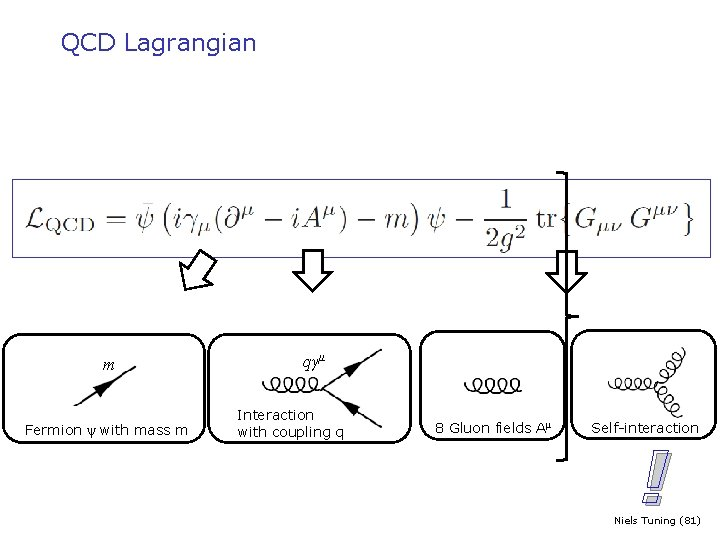QCD Lagrangian m Fermion ψ with mass m qγμ Interaction with coupling q 8 Gluon fields Aμ Self-interaction ! Niels Tuning (81)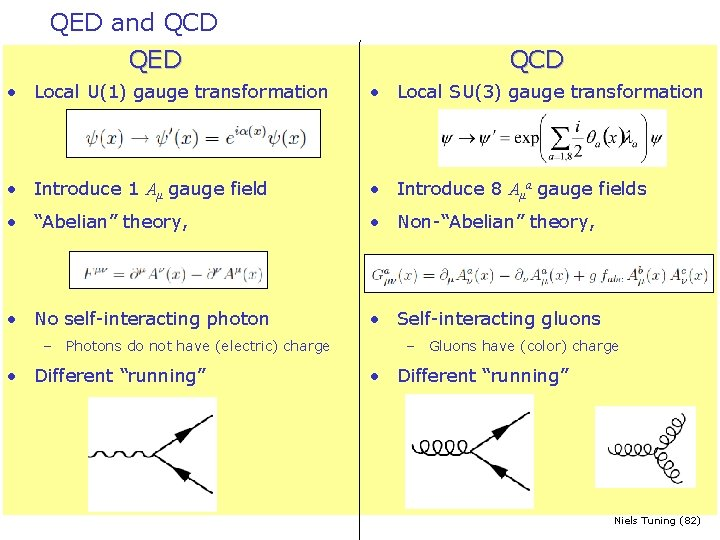QED and QCD QED QCD • Local U(1) gauge transformation • Local SU(3) gauge transformation • Introduce 1 Aμ gauge field • Introduce 8 Aμa gauge fields • “Abelian” theory, • Non-“Abelian” theory, • No self-interacting photon • Self-interacting gluons – Photons do not have (electric) charge • Different “running” – Gluons have (color) charge • Different “running” Niels Tuning (82)Which symmetries do we impose ? Rotations in hypercharge space Rotations in color space Rotations in weak isospin space For example SU(2)L: 2 x 2 complex matrices (det=1) 3 basis-rotations 3 vector fields QED: U(1)Y 1 degree of freedom: Weak Force: SU(2)L 3 degrees of freedom: Strong Force: SU(3)C 8 degrees of freedom: γ W+, W- en Z 0 8 gluons All spin-1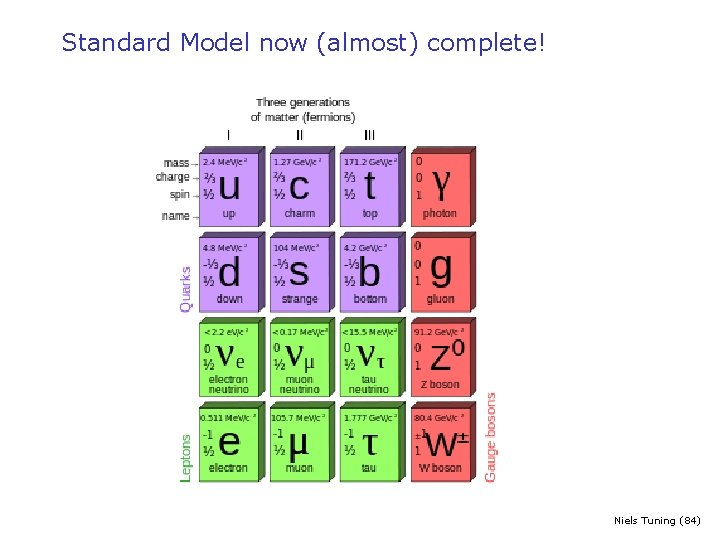Standard Model now (almost) complete! Niels Tuning (84)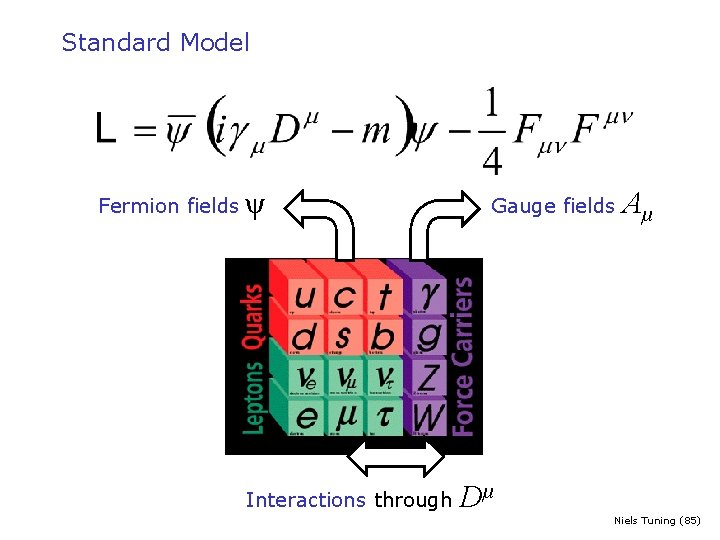Standard Model Fermion fields ψ Interactions through Gauge fields Dμ Aμ Niels Tuning (85)Standard Model Prof. dr. J. Ellis Niels Tuning (86)Standard Model Todo-list: • e+e- scattering – QED at work (LEP): R, neutrinos • e+p scattering – QCD at work (HERA): DIS, structure functions, scaling • No masses for W, Z – (LHC/ATLAS) Higgs mechanism, Yukawa couplings • Consequences of three families – (LHC/LHCb) CKM-mechanism, CP violation Niels Tuning (87)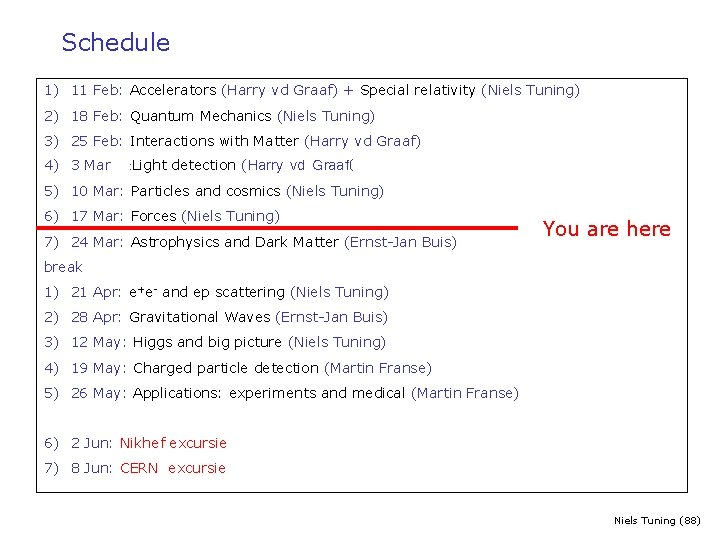Schedule 1) 11 Feb: Accelerators (Harry vd Graaf) + Special relativity (Niels Tuning) 2) 18 Feb: Quantum Mechanics (Niels Tuning) 3) 25 Feb: Interactions with Matter (Harry vd Graaf) 4) 3 Mar : Light detection (Harry vd Graaf( 5) 10 Mar: Particles and cosmics (Niels Tuning) 6) 17 Mar: Forces (Niels Tuning) 7) 24 Mar: Astrophysics and Dark Matter (Ernst-Jan Buis) You are here break 1) 21 Apr: e+e- and ep scattering (Niels Tuning) 2) 28 Apr: Gravitational Waves (Ernst-Jan Buis) 3) 12 May: Higgs and big picture (Niels Tuning) 4) 19 May: Charged particle detection (Martin Franse) 5) 26 May: Applications: experiments and medical (Martin Franse) 6) 2 Jun: Nikhef excursie 7) 8 Jun: CERN excursie Niels Tuning (88)Plan 1900 -1940 1945 -1965 -1975 1) Intro: Standard Model & Relativity 11 Feb 2) Basis 18 Feb 1) Atom model, strong and weak force 2) Scattering theory 3) Hadrons 1) Isospin, strangeness 2) Quark model, GIM 4) Standard Model 1) QED 2) Parity, neutrinos, weak inteaction 3) QCD 10 Mar 17 Mar 1975 -2000 5) e+e- and DIS 21 Apr 2000 -2015 6) Higgs and CKM 12 May Niels Tuning (89)